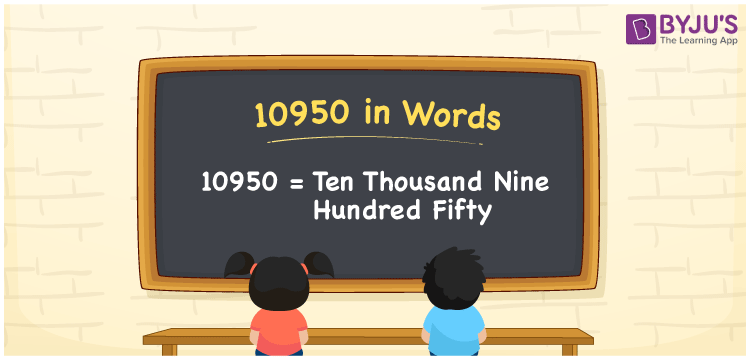# 10950 in Words

We can write 10950 in words as Ten thousand nine hundred fifty. It is possible to easily derive the number 10950 in words using a place value chart. If you listed 10950 names in a notebook, you could say, “I listed Ten thousand nine hundred fifty names in the notebook”. Thus, we use the word form of the number to express the given quantity or measure. In this article, you will learn how to write 10950 in words, along with some facts about the number 10950.

 10950 in words Ten thousand nine hundred fifty Ten thousand nine hundred fifty in Numbers 10950

## 10950 in English Words

Generally, we express numbers in words using the English alphabet and thus, we spell 10950 in English words as “Ten thousand nine hundred fifty”.## How to Write 10950 in Words?

The number 10950 has five digits. Thus, we need to make a place value chart with 5 columns so that each of these five digits can be assigned a specific place value. This can be observed from the table below.

 Ten thousands Thousands Hundreds Tens Ones 1 0 9 5 0

Here, ones = 0, tens = 5, hundreds = 9, thousands = 0, ten thousands = 1

So, 1 × Ten thousand + 0 × Thousand + 9 × Hundred + 5 × Ten + 0 × One

= 1 × 10000 + 0 × 1000 + 9 × 100 + 5 × 10 + 0 × 1

= 10000 + 900 + 50

= Ten Thousand + Nine hundred + Fifty

= Ten thousand nine hundred fifty

10950 is a natural number that is the successor of 10949 and predecessor of 10951.

10950 in words – Ten thousand nine hundred fifty

Is 10950 an odd number? – No

Is 10950 an even number? – Yes

Is 10950 a prime number? – No

Is 10950 a composite number? – Yes

Is 10950 a perfect square number? – No

Is 10950 a perfect cube number? – No

## Frequently Asked Questions on 10950 in Words

### How do you write 10950 in words?

We can write 10950 in words as “Ten thousand nine hundred fifty”.

### How to write Rs. 10950 in words on a cheque?

On a cheque, we generally write Rs. 10950 in words as “Ten thousand nine hundred fifty rupees only”.

### Express the answer for 10950 – 9855 in words.

10950 – 9855 = 1095 Therefore, the value of 10950 – 9855, i.e., 1095 in words is One thousand ninety-five.